# Question 9 0.1 pts A monopsonist has the production function Q=4.1 and faces the following labor supply and product dem...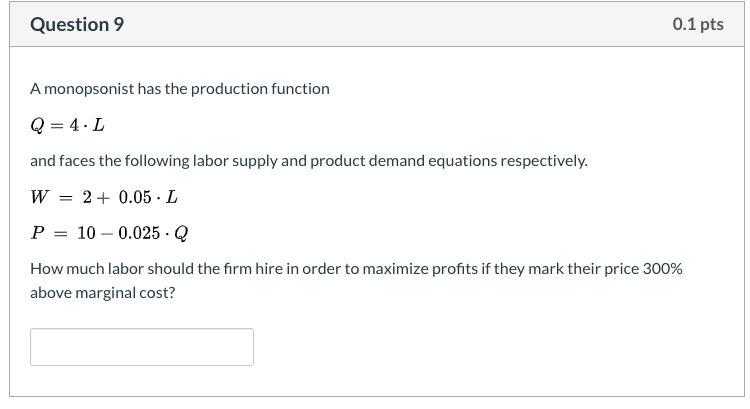Question 9 0.1 pts A monopsonist has the production function Q=4.1 and faces the following labor supply and product demand equations respectively. W = 2 + 0.05L P = 10 – 0.025.Q How much labor should the firm hire in order to maximize profits if they mark their price 300% above marginal cost?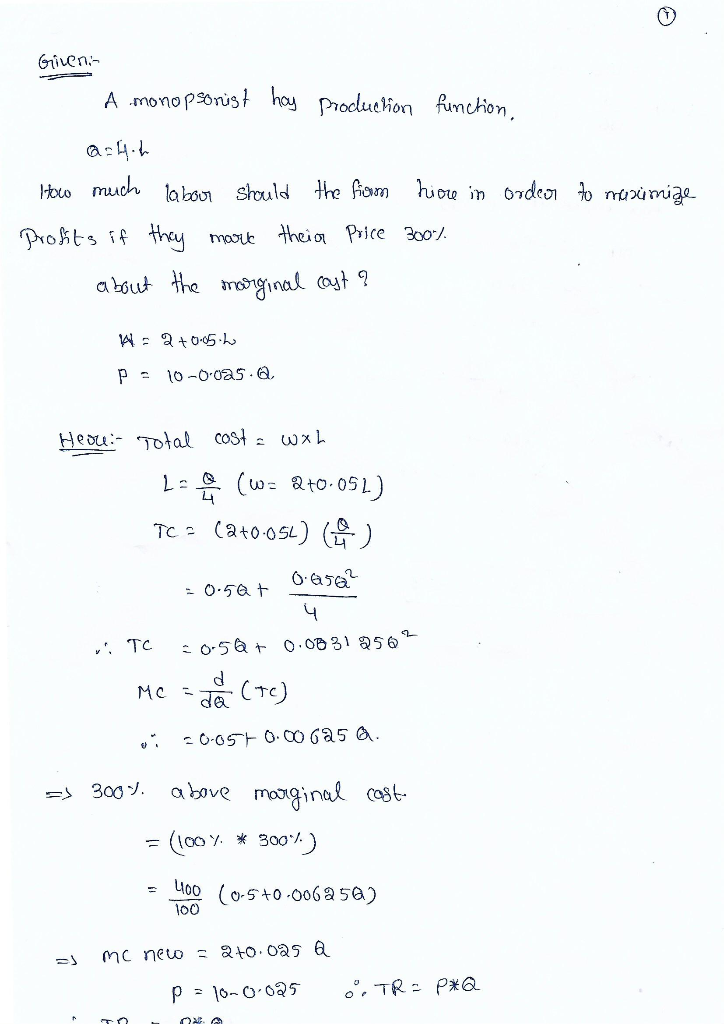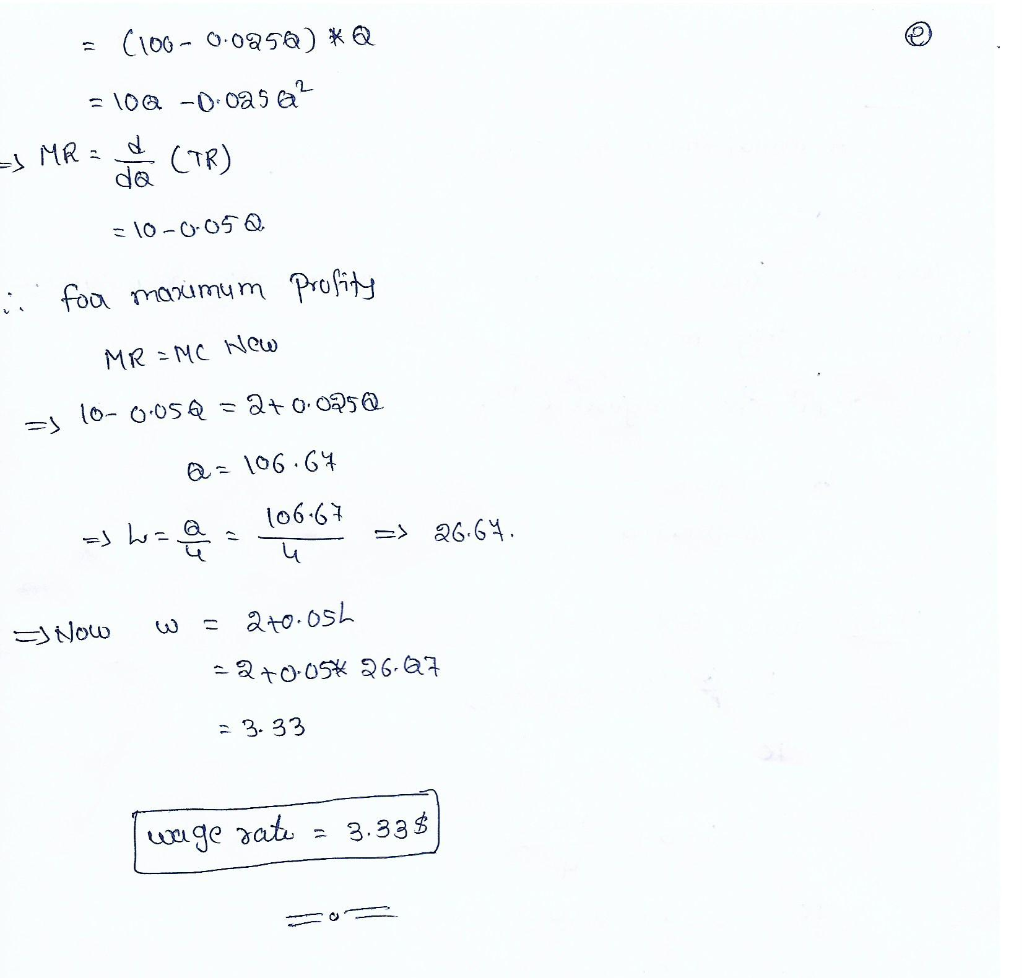##### Add Answer of: Question 9 0.1 pts A monopsonist has the production function Q=4.1 and faces the following labor supply and product dem...
Similar Homework Help Questions
• ### ect Question 10 0/0.1 pts A monopsonist has the production function Q = 4.1 and faces the following labor supply an...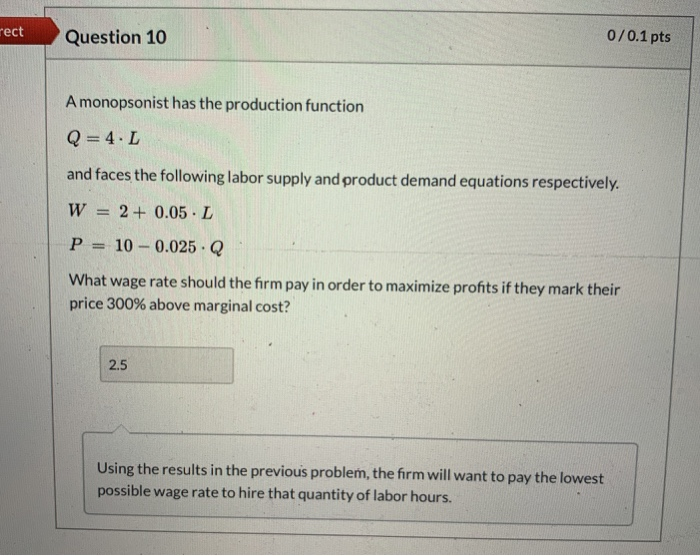ect Question 10 0/0.1 pts A monopsonist has the production function Q = 4.1 and faces the following labor supply and product demand equations respectively. W = 2 + 0.05 L P = 10 -0.025 Q What wage rate should the firm pay in order to maximize profits if they mark their price 300% above marginal cost? 2.5 Using the results in the previous problem, the firm will want to pay the lowest possible wage rate to hire that quantity...

• ### 0/0.1 pts Incorrect Question 9 A monopsonist has the production function Q=4.L and faces the foflowing...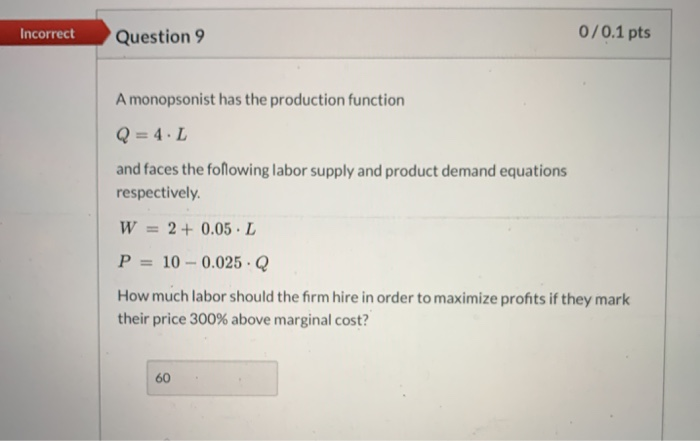0/0.1 pts Incorrect Question 9 A monopsonist has the production function Q=4.L and faces the foflowing labor supply and product demand equations respectively W= 2+ 0.05 L P= 10-0.025 Q How much labor should the firm hire in order to maximize profits if they mark their price 300% above marginal cost? 60

• ### please i need the answers. the questions are related, you need to find questions 9 then...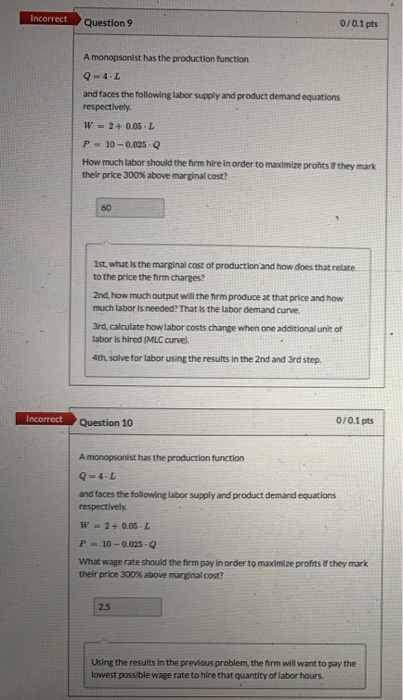please i need the answers. the questions are related, you need to find questions 9 then 10 Incorrect Question 9 0/0.1 pts A monopsonist has the production function Q = 4. and faces the following labor supply and product demand equations respectively. W = 2+ 0.05.L P 10 -0.025 How much labor should the firm hire in order to maximize profits if they mark their price 300% above marginal cost? 1st, what is the marginal cost of production and how...

• ### A monopsonist faces the following demand curve for their product: P = 20 - 0.005 XQ...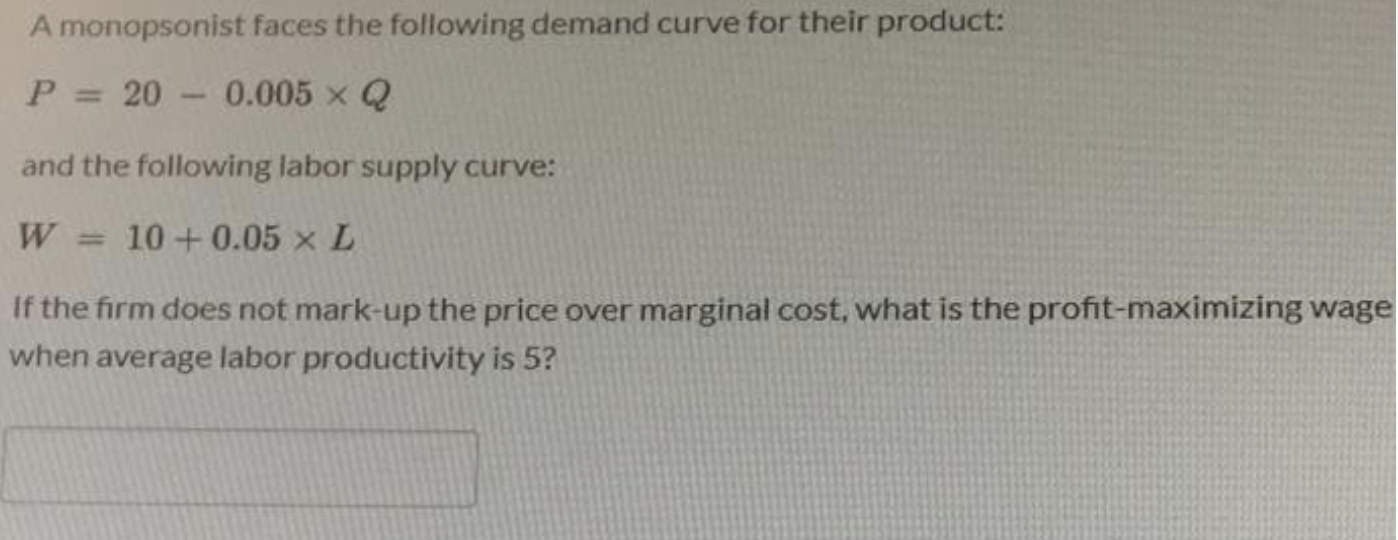A monopsonist faces the following demand curve for their product: P = 20 - 0.005 XQ and the following labor supply curve: W = 10+ 0.05 x L If the firm does not mark-up the price over marginal cost, what is the profit-maximizing wage when average labor productivity is 5?

• ### Consider the production function Q = 6L^2 - L^3.

4. Consider the production function Q = 6L^2 - L^3.a. Find average product. Find the number of labor (L) that the firm should hire to maximize average product.b. Find marginal product. Find the number of labor (L) that the firm should hire to maximize marginal product.c. Find the number of labor (L) that the firm should hire to maximize total product of labor.d. Find the number of labor (L) that the firm has zero average product.

• ### Imagine a firm operating in competitive labor and product markets. The market price of Q is...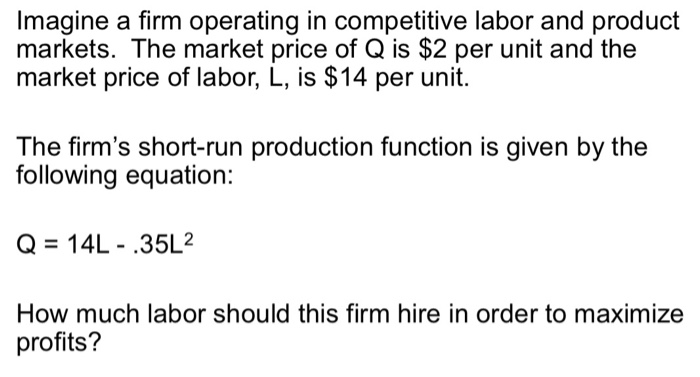Imagine a firm operating in competitive labor and product markets. The market price of Q is \$2 per unit and the market price of labor, L, is \$14 per unit. The firm's short-run production function is given by the following equation: Q = 14L - .35L2 How much labor should this firm hire in order to maximize profits?

• ### A monopolist faces the following demand curve: Q = 260-2P Where Q is the weekly production...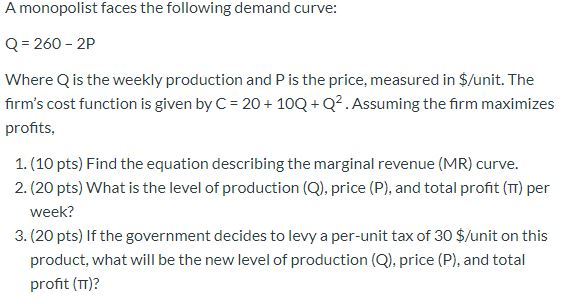A monopolist faces the following demand curve: Q = 260-2P Where Q is the weekly production and P is the price, measured in \$/unit. The firm's cost function is given by C= 20 + 10Q+Q2. Assuming the firm maximizes profits, 1. (10 pts) Find the equation describing the marginal revenue (MR) curve. 2. (20 pts) What is the level of production (Q), price (P), and total profit (TT) per week? 3. (20 pts) If the government decides to levy a...

• ### 2. Consider a firm producing pizza with production function q = KL, that faces input prices...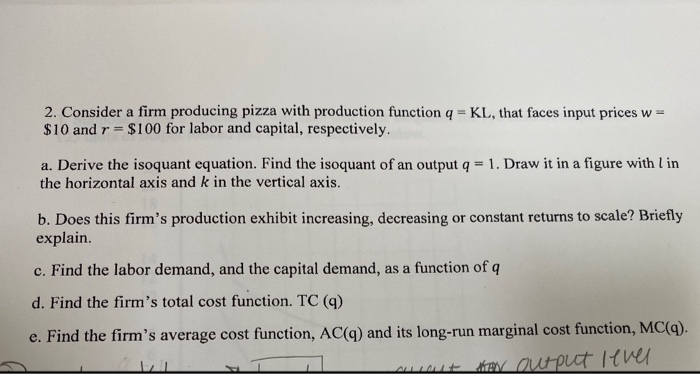2. Consider a firm producing pizza with production function q = KL, that faces input prices w= \$10 and r = \$100 for labor and capital, respectively. a. Derive the isoquant equation. Find the isoquant of an output q = 1. Draw it in a figure with l in the horizontal axis and k in the vertical axis. b. Does this firm's production exhibit increasing, decreasing or constant returns to scale? Briefly explain c. Find the labor demand, and the...

• ### Can someone help with the problem below? Suppose a firm's production function is y = 3L...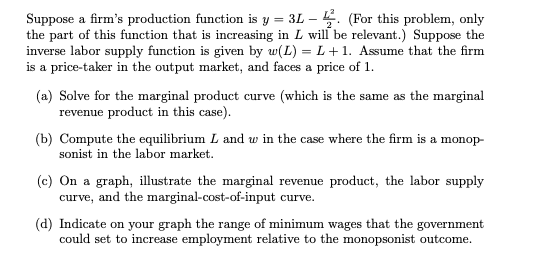Can someone help with the problem below? Suppose a firm's production function is y = 3L - (For this problem, only the part of this function that is increasing in L will be relevant.) Suppose the inverse labor supply function is given by w(L) = L + 1. Assume that the firm is a price-taker in the output market, and faces a price of 1. (a) Solve for the marginal product curve (which is the same as the marginal revenue...

• ### Suppose a firm has the following production function: Q = 2K L. The marginal product of...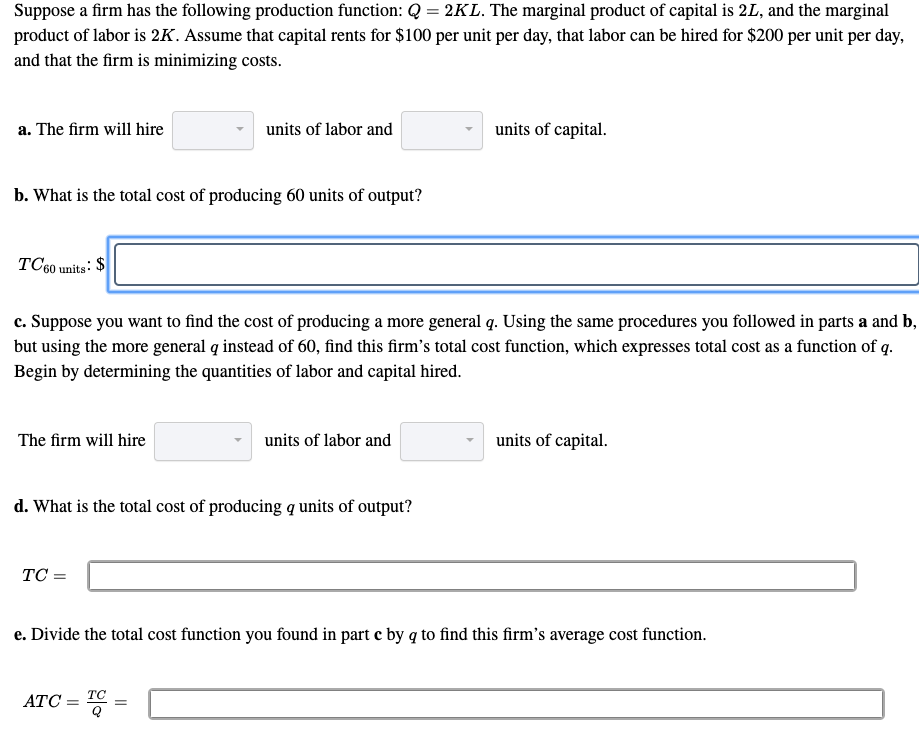Suppose a firm has the following production function: Q = 2K L. The marginal product of capital is 2L, and the marginal product of labor is 2K. Assume that capital rents for \$100 per unit per day, that labor can be hired for \$200 per unit per day, and that the firm is minimizing costs. a. The firm will hire units of labor and - units of capital. b. What is the total cost of producing 60 units of output?...

Free Homework App